Analysis of Unsteady MHD Thin Film Flow of a Third Grade Fluid with Heat Transfer down an Inclined Plane | OMICS International
Journal of Applied & Computational Mathematics

# Analysis of Unsteady MHD Thin Film Flow of a Third Grade Fluid with Heat Transfer down an Inclined Plane

Aiyesimi YM1, Okedayo GT2 and Lawal OW1*

1Department of Mathematics, Federal University of Technology Minna, Niger State, Nigeria

2Department of Mathematics, Ondo State University of Science and Technology Okitipupa, Ondo State, Nigeria

*Corresponding Author:
Lawal OW
Department of Mathematics
Federal University of Technology Minna
Niger State, Nigeria
Tel: 07032270305, 234-(0)7098809476
E-mail: [email protected]

Received Date: January 17, 2014; Accepted Date: February 12, 2014; Published Date: February 17, 2014

Citation: Aiyesimi YM, Okedayo GT, Lawal OW (2014) Analysis of Unsteady MHD Thin Film Flow of a Third Grade Fluid with Heat Transfer down an Inclined Plane. J Appl Computat Math 3:152. doi: 10.4172/2168-9679.1000152

Copyright: © 2014 Aiyesimi YM, et al. This is an open-access article distributed under the terms of the Creative Commons Attribution License, which permits unrestricted use, distribution, and reproduction in any medium, provided the original author and source are credited.

Visit for more related articles at Journal of Applied & Computational Mathematics

#### Abstract

An investigation is made for unsteady MHD thin film flow of a third grade fluid down an inclined plane with no slip boundary condition. The governing nonlinear partial differential equations involved are reduced to linear partial differential equations using regular perturbation method. The resulting equations were solved using method of separation of variable and eigen functions expansion. The solutions obtained were examined and discussed graphically. It is interesting to find that the variation of the velocity and temperature profile with the magnetic field parameter and gravitational parameter depends on time.

#### Keywords

Eigen value; MHD thin film; Homotopy

#### Introduction

recent years considerable interest has been developed in the study of the flow of non- Newtonian fluid down an inclined plane because of its important applications in science, engineering and technology. Examples of their application can be found in ink-jet print, polymer processing, silvering processing, plasma enhanced chemical vapour deposition as well as in magnetorheological thin film deposition. Because of the complexity of non-Newtonian fluids, it is very difficult to analyse a single model that exhibits all its properties. The normal stress differences is describe in second grade of non-Newtonian fluid, but it cannot predict shear thinning or thickening properties due to its constant apparent viscosity. The third grade fluid model attempt to include such characteristics of viscoelastics fluids. Many authors have successfully analysed the nonlinear differential equation governing the flow of a third grade fluid [1-3]. He [4-6] introduced a new perturbation method which is a combination of regular perturbation method and homotopy as used in topology. Siddiqui et al. , Khan and Mahmood , Aiyesimi et al.  and other authors recently applied this newly introduced homotopy perturbation method to solved nonlinear differential equation that governing the steady thin film flow of a third grade fluid down an inclined plane.

In this paper, we obtain analytical solution of the unsteady MHD thin film flow problem for a third grade fluid down an inclined plane with no slip boundary condition as formulated in Aiyesimi et al. . The governing nonlinear differential equations involved are reduced to linear differential equations using regular perturbation method. The resulting equations were solved using method of separation of variable and eigen function expansion.

#### Problem Formulation

In Aiyesimi et al. , we studied MHD thin film flow of a third grade fluid down an inclined plane. We assumed that the flow was unsteady, the ambient air was stationary, surface tension negligible and the thin film is of uniform thickness δ [11-15]. The whole system was subjected to magnetic field and in the absence of pressure gradient, we obtained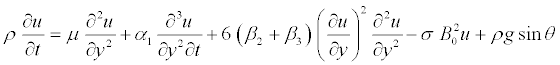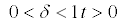(1)

with the boundary condition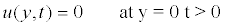(2)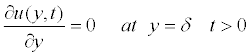(3)

and initial condition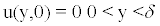(4)

where ρ is the density of the fluid, u is the fluid velocity, B0 represent induced magnetic field, σ is the electrical conductivity, α1, β2 and β3 are the material constant and θ indicate angle of inclination.

The energy equation for the thermodynamically compatible third grade fluid with viscous dissipation, work done due to deformation and Joule heating was given as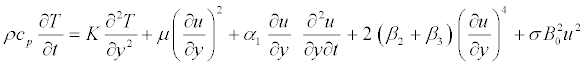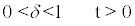(5)

with boundary condition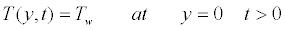(6)

and initial condition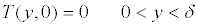(7)

where cp and k are respectively, specific heat capacity and thermal conductivity of the fluid. T is the temperature of the fluid, Tw is the temperature of the plane and Tδ is the temperature of the ambient fluid.

We define the following non-dimensional quantities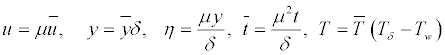(8)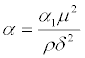, is the second grade parameter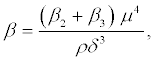is the third grade parameter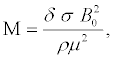is the magnetic parameter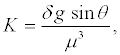is the gravitational parameter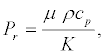is the Prandth number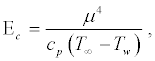is the Eckert number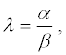is the fluid grade ratio

In terms of these above non-dimensional variables and parameters, equation (1)-(8) are written as (as we dropped “hats” for convenience)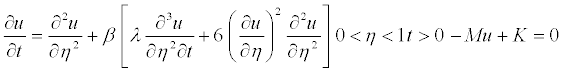(9)

with the boundary and initial condition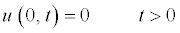(10)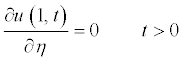(11)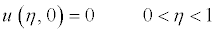(12)

For energy equation, we have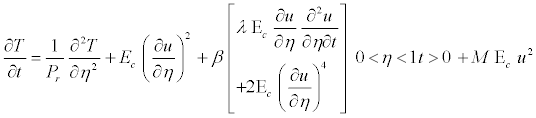(13)

with the boundary and initial condition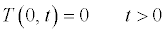(14)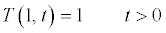(15)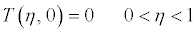(16)

#### Solutions of the Problem

The basic requirement for the application of solution by perturbation method is the existence of small or large parameter in equation (10), therefore let assumed ε = β as a small parameter and expand u(η,t) in the Poincare-type series of the form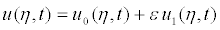(17)

Substituting equation (18) into equation (10) – (13) and collect the like terms base on the power of , we have

Zeroth–order problem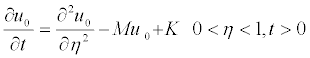(18)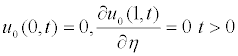(19)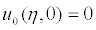,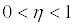(20)

First order problem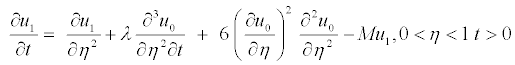(21)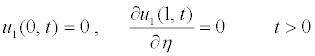(22)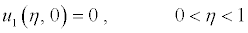(23)

In order to solve equation (19) which is Initial Boundary Value Problem (IBVP) together with equation (20) and (21), let assume a solution of the form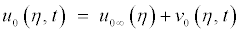(24)

where u0∞ is the equilibrium solution of equation (19)–(21) which satisfies the Boundary Value Problem (BVP)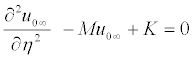(25)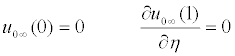(26)

The solution of equation (26) with boundary condition (27) is given as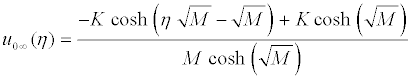(27)

Substituting equation (28) into (25) to give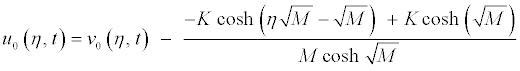(28)

also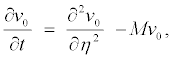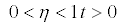(29)

The boundary and initial condition (20) and (21) becomes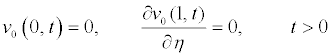(30)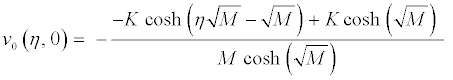(31)

Equation (30) and boundary condition (31) are linear and homogeneous, which have a solution of the form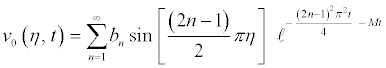(32)

Substituting equation (28) and (33) into equation (25) to gives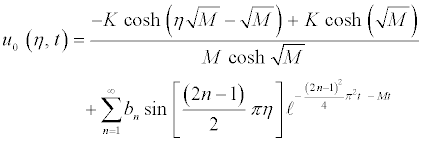(33)

where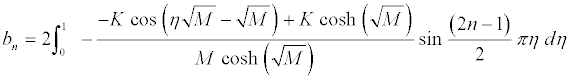Solving first order problem, equation (22) can written as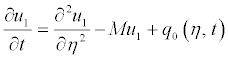,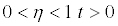(34)

where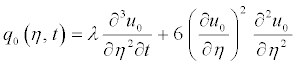The eigenvalues and eigenfunctions of the corresponding homogeneous problem of equation (35) when q0(η,t)= 0 are respectively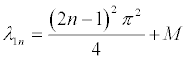(35)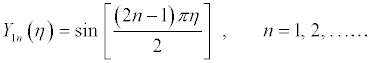(36)

Since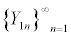is a complete sets, we may consider for the solution of equation (35) an expansion of the form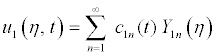(37)

substituting equation (33) and (38) into (18) to obtain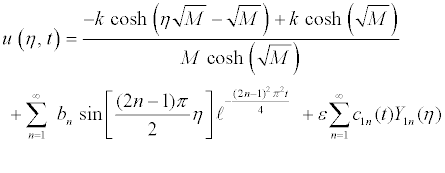(38)

Therefore when n=1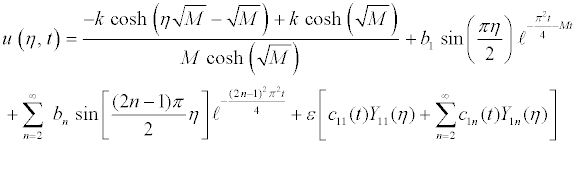(39)

where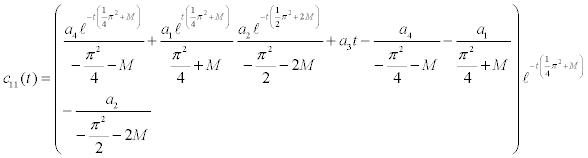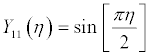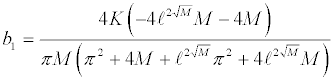For n=2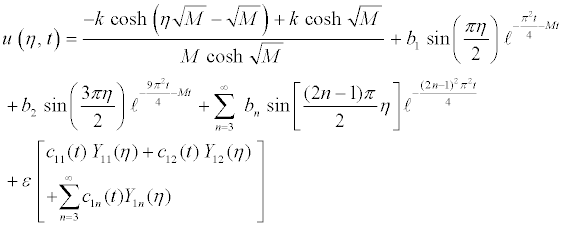(40)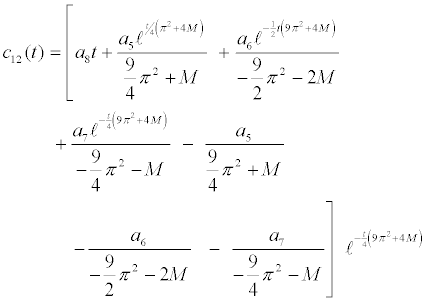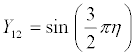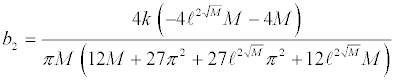where i a ( i =1,2,...8 ) are constant

Next, we find the approximate solution for energy equation (14) with boundary and initial condition (15)–(17) by chosen u(η,t) from equation (40) when n=1 and ε which is equal to β is turn to zero (i.eε = β →0 ) as temperature increases.

Therefore equation (14) becomes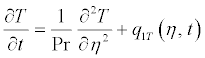;(41)

where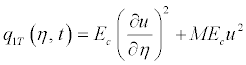also, we choose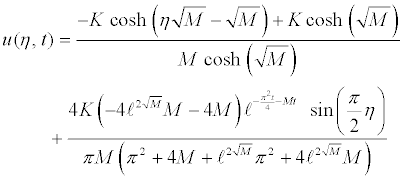(42)

Let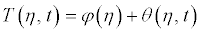(43)

Substituting (45) into (42), (15) - (17) to obtain the following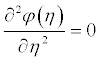(44)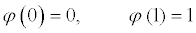(45)

also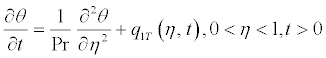(46)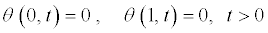(47)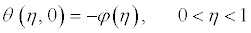(48)

Solving equation (46) with (47), we have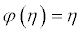(49)

To solve equation (48) together with (49)–(51), the eigenvalues and eigenfunctions of the corresponding homogeneous problem of equation (48) when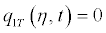are respectively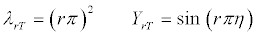(50)

where r =1, 2, . . .

Since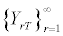is a complete sets, we may consider for the solution an expansion of the form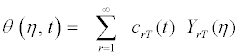(51)

when r=1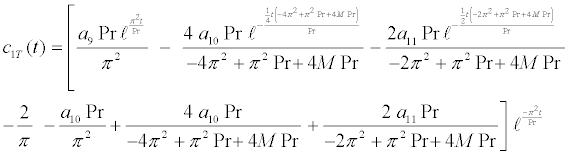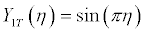Therefore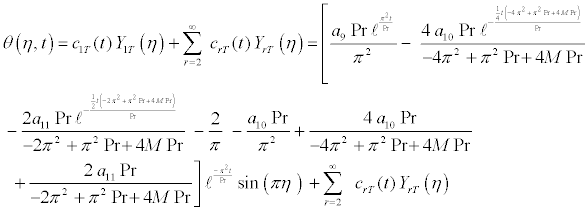(52)

Also, when r=2, then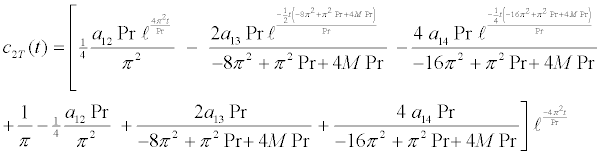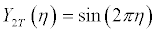Then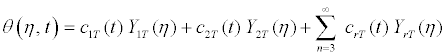(53)

Therefore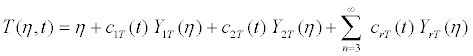(54)

Again, when n=2 and ε = β →0 , we choose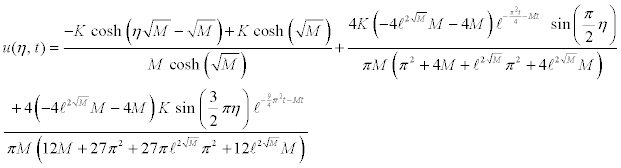(55)

then we obtained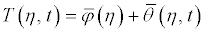(56)

where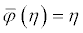(57)

and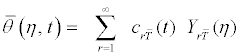(58)

when r=1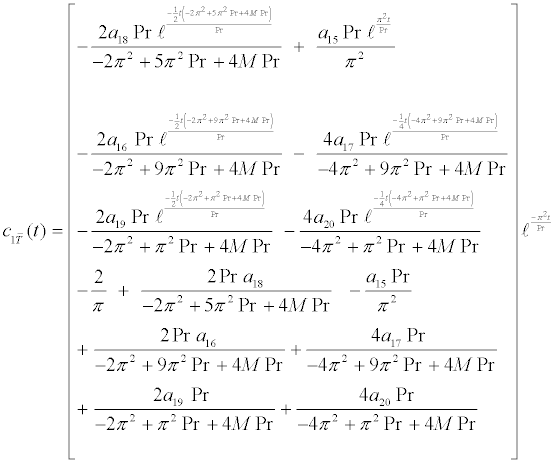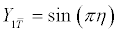, when r=2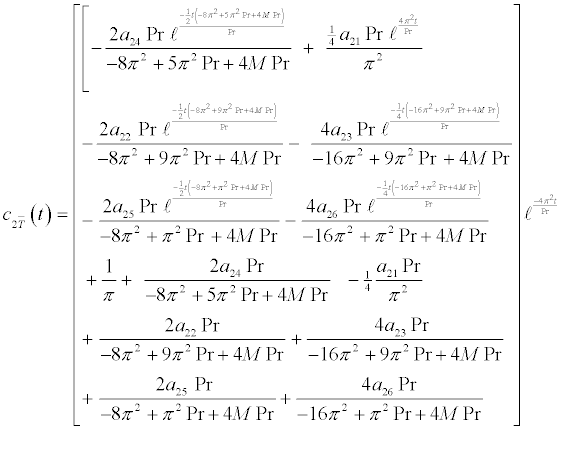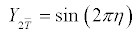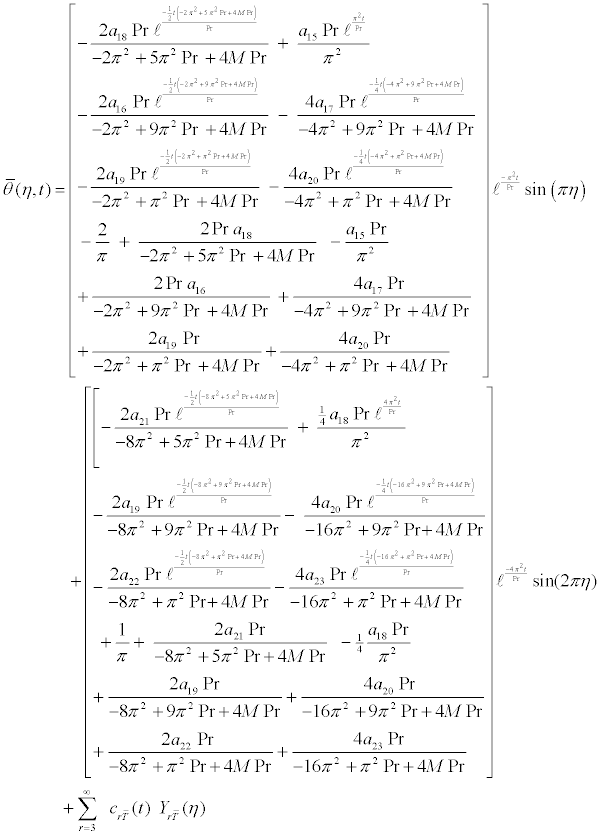and αi (i=9,10,11,....,24) are constant and can be calculated through simple computation.

#### Results and Discussion

In this section, we discuss the behaviour of the velocity and temperature profile u and T of unsteady MHD thin film flow of a third grade fluid as it move down an inclined plane in the absence of slip parameter.

Figure 1a-1d and Figure 2a-2d shows the time development of velocity profile u and temperature profile T for various value of time t and for M=1,5,10 and 25.

Figure 1: Time development of velocity profile for various values of M and time t when K=1 and λ = 0.1 .

Figure 2: Time development of temperature profile T for various values of M and time t when K=1, λ = 0.1 , Pr=1 and Ec=1.

Figure 1 is evaluated for K =1, λ = 0.1 and β =ε = 0.001. It is found that velocity profile decrease with increases in magnetic parameter M at large time but increases them at small time. Also it is noticed that at a fixed value of M, u increases with time for short period and then decreases as time develops. However, at large value of M, u attain its steady state monotonically with time as shown in Figure 1d.

Figure 2 is evaluated for K =1, λ = 0.1, β = 0, Pr =1 and Ec =1. It is observed that T slightly increases with time for short period and then remains steady as time developed for various values of M. This clearly indicates that the purpose of introducing magnetic field is to decrease the viscous and Joule dissipation as a result of decrease in the velocity profile u and its gradient.

Figures 3 and 4 shows the time development of the velocity and temperature u and T respectively for various values of time t and for gravitational parameter K=1,2,3 and 4. There figure are plotted using M = 5, λ = 0.1,Pr =1 and Ec =1. It is observed from Figure 3a-3d that for all value of K, u increases at small time but decreases at large time. Also it is shown that the rate at which increases for a short period and the rate at which it decreases as the time develop is the same for each particular value of . This clearly revealed that the effect of gravitational parameter on velocity K depend only on gravitational force and angle of inclination but not on time .

Figure 3: Time development of velocity profile u for various values of K and time t when M=5 and λ = 0.1.

Figure 4: Time development of temperature profile T for various values of K and time t when M=5, λ = 0.1 , Pr=1 and Ec=1.

Figure 4a-4d indicate that the effect of the parameter K on temperature profile T depend not only gravitational force, angle of inclination and magnetic field but also on time t. At a small values of K it is observed that T increases slightly at small time but remain steady as time develops. However, when K increase as a result of increase in angle of inclination, T increase substantially at small time and slightly increase at large time. And the rate of increment in T is higher in short period than as time develops for small value of M.

This can be attributed to the fact that, for small times, an increase in K increases u largely thereby increase Joule and viscous dissipation at small value of M. But at large value of M and increase in K decrease u largely as time develops thereby decreases Joule and viscous dissipation and make T to remain steady as illustrated in Figure 5a-5d.

Figure 5: Time development of temperature profile T for various values of K and time t when M=20, λ = 0.1 , Pr=1 and Ec=1.

#### Conclusion

The unsteady MHD thin film flow of a third grade fluid down an inclined plane with heat transfer has been studied in the presence of a uniform magnetic field. The effect of magnetic field parameter and gravitational parameter on the velocity and temperature profile has been investigated. It is confirmed that for any value of magnetic field parameter and gravitational parameter, it has a mark effect on steady and unsteady state time of both the velocity and temperature profile. Also, it is interesting to find that the variation of the velocity and temperature profile with the magnetic field parameter and gravitational parameter depends on time.

#### References

Select your language of interest to view the total content in your interested language

### Article Usage

• Total views: 12078
• [From(publication date):
March-2014 - Dec 13, 2019]
• Breakdown by view type
• HTML page views : 8259Can't read the image? click here to refresh